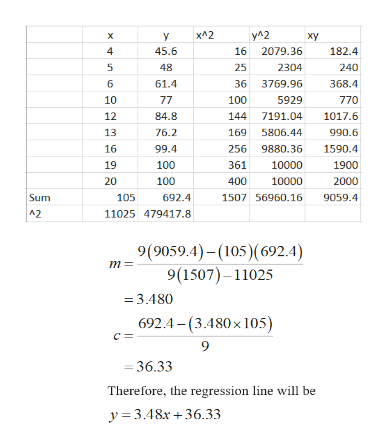# Run a regression analysis on the following data set, where yyis the final grade in a math class and xxis the average number of hours the student spent working on math each week. hours/weekxGradey445.6548661.4107710771284.81376.21699.41910020100State the regression equation y=m⋅x+by=m⋅x+b, with constants accurate to two decimal places. What is the predicted value for the final grade when a student spends an average of 15 hours each week on math? Grade = Round to 1 decimal place.

Question
26 views

Run a regression analysis on the following data set, where
y
y
is the final grade in a math class and
x
x
is the average number of hours the student spent working on math each week.
hours/week
x
y
4
45.6
5
48
6
61.4
10
77
10
77
12
84.8
13
76.2
16
99.4
19
100
20
100

State the regression equation
y
=
m

x
+
b
y=m⋅x+b
, with constants accurate to two decimal places.

What is the predicted value for the final grade when a student spends an average of 15 hours each week on math?
Grade = Round to 1 decimal place.

check_circle

Step 1

According to the provided information, the final grade in a math class and the number of hours the student spent working on math each week are given.

Here, Number of hours is independent variable (x) and Grade is dependent variable (y)

The regression line can be expressed as:

Step 2

The regression coefficient can be calculated as:

Step 3

The required data can b...help_outlineImage Transcriptioncloseх^2 yA2 х y ху 4 45.6 16 2079.36 182.4 5 48 25 2304 240 36 3769.96 6 61.4 368.4 10 77 100 5929 770 84.8 12 144 7191.04 1017.6 76.2 169 5806.44 990.6 13 99.4 256 9880.36 16 1590.4 19 100 361 10000 1900 20 100 400 10000 2000 Sum 2 105 692.4 1507 56960.16 9059.4 11025 479417.8 9(9059.4)-(105)(692.4) 9(1507)-11025 m= = 3.480 692.4-(3.480x 105) с: 9 36.33 Therefore, the regression line will be y3.48x+36.33 n fullscreen

### Want to see the full answer?

See Solution

#### Want to see this answer and more?

Solutions are written by subject experts who are available 24/7. Questions are typically answered within 1 hour.*

See Solution
*Response times may vary by subject and question.
Tagged in

### Other